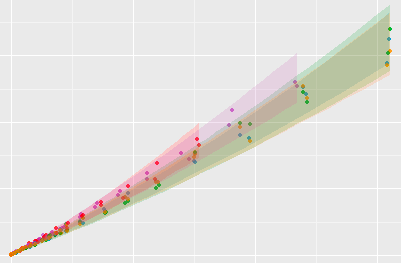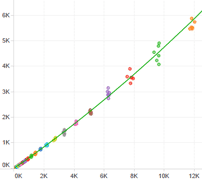In this days of big data world, situations where you have have a large amount of data from which you don't have to "read between lines" but "read between the numbers" arises more often and naturally. For me, it is a fascinating subject, sometimes a little bit frustrating or challenging because I don't have all the required skills and can take me some of time to clean, reshape and digest the data.

I am trying to learn how to be a "datactive". As I don`t have a great memory, any time I have a challenge in this subject, forcing me to do a little "research" or to peruse some books, I write an article in this section, with enough bread crumbs to help me to come back over those steps when required.

# Latest Data Analysis Articles

Posted:
Last edited:
<p>Aquí queremos mostrar los datos que describen la evolución y la situación actual de la pandemia en el Perú, así como proveer algunos indicadores clave, señalando cuanto y qué tan rápido se esparce, con detalle de nuestros departamentos del Perú y con referencias a otros países para comprender mejor los datos propios. Como siempre, la mejor manera de entender los datos es a través de gráficos.</p> <p>Estos gráficos e indicadores van a ser actualizados en lo posible tan pronto como hay nuevos datos.</p>
Posted:
Last edited:<p>In a previous article we introduced a <em>"A Simple DSLR Camera Sensor Noise Model"</em> and then we used this model to analyze a camera sensor raw noise in <em>"Use of Linear Regressions to Model Camera Sensor Noise"</em>. Those articles deal with the theoretical and practical basis to analyze noise from raw image samples.</p> <p>The input to that analysis are samples of photographs taken at different exposition levels, from which we compute the variance and mean of the photosite readings. Until now, we have prepared the samples and computed their variance and mean using <em>Iris Software</em>. But that workflow was very labor intensive, error prone, and inhibit us to further analysis like the study of the covariance between channels in the same sample because <em>Iris software</em> does not bring that information. Furthermore, <em>Iris</em> is a windows only software, which prevents users with other OSs to collect noise data using this tool.</p> <p>To solve those issues, we have prepared a R package called <code>imgnoiser</code> (from IMaGe NOISE R package). In this article, we introduce you to its usage, which automatizes the acquisition, analysis of noise statistics, letting us to focus in the study, instead of devoting a lot of time to collect the data or to prepare code to study that data.</p> <p>You can install the <code>imgnoiser</code> package from github using <code>devtools::install_github('oscardelama/imgnoiser')</code>...</p>
Posted:
Last edited:
<p>This is the repository of the formulas we use along other articles.</p> <p>Currently contains formulas for:</p> <ul> <li><p>Statistics</p> <ul> <li>Expected Value</li> <li>Covariance</li> <li>Variance</li> <li>Standard Deviation</li> <li>Correlation Coefficient</li> </ul></li> <li><p>Analytic Relationship of two Numerically Correlated Functions</p> <ul> <li>Linear Case</li> <li>Quadratic case</li> </ul></li> </ul>
Posted:
Last edited:
<p>Notes about Linear Regression<br /></p> <p>These are my personal notes related about how to fit Linear Regression, how to interpret the most useful R tests. </p>
Posted:
Last edited:<p>In the post <em>"Use of Linear Regressions to Model Camera Sensor Noise"</em> we need to fit two linear regressions. However, it turns out that fitting those regressions was not a trivial task: the data was <em>heteroscedastic</em> (with non constant variance) and required some analysis, transformation and cleaning.</p> <p>This was a chance to learn and practice some techniques about linear regressions using the <em>R Language</em>. This article details all the steps, considerations and tools we used to fit a valid model over that heteroscedastic data.</p>